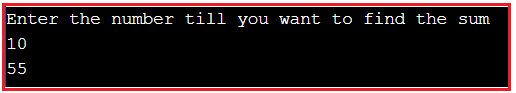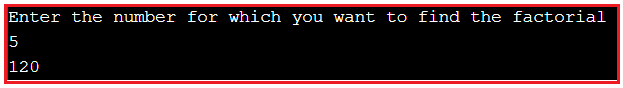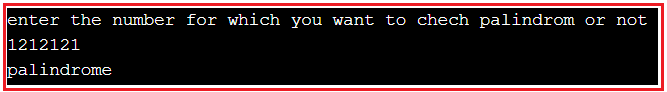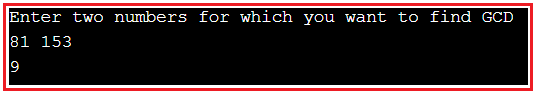# Assignment Solution of Loops

## Assignment Solution of Loops in C++

In this article, I am going to give you the solutions that we give you as an assignment in our Loops in C++ article. So, please read our Loops in C++ article, if you have not read it yet. First, try to solve the problem by yourself and then only look at the below solutions.

##### Program to find the sum of first N natural numbers.

Testcase1: input: N=20
Expected output=210
Note: Also select which loop is better to find the first N natural numbers.
Solution: To make a choice of which loop? Let’s understand the question. Here we need to find the sum of N natural numbers.

Let’s say we want to find some of the first 5 numbers. 1,2,3,4,5 sum of these numbers is 1+2+3+4+5. Here I know how many times I need to run the loops. Yes. You guessed it right 5 times as we have 5 numbers. As discussed in our previous article, if we know how many times to execute then the best choice is counter loops (for-loop). However; you can implement it using other loops as well.

```#include <iostream>
using namespace std;
int main ()
{
int sum = 0;
int number;
cout << "Enter the number till you want to find the sum" << endl;
cin >> number;
for (int i = 1; i <= number; ++i)
{
sum += i;
}
cout << sum;
return 0;
}
```

Output:##### Program to find factorial of a number.

Testcase1: input N=5;
Output=120;

Solution:
Algorithm for finding factorial of a number in C++
1. Declare variables i (for loop) and fact (for storing final answer).
2. Initialize fact with value 1
3. Take input from the user whose factorial u want to find (suppose n here)
4. Run a loop from i=n to i>0
for(i=n;i>0;i – -)
fact=fact*i;
5. Print fact on the console window

```#include <iostream>
using namespace std;
int main ()
{
int fact = 1;
int number;
cout << "Enter the number for which you want to find the factorial" << endl;
cin >> number;
for (int i = number; i > 0; --i)
{
fact *= i;
}
cout << fact;
return 0;
}
```

Output:##### Program to find a palindrome or not.

Testcase1: input:1212121
Output: palindrome
Solution:

```#include <iostream>
using namespace std;
int main ()
{
int number, res = 0, digit = 0;
cout << "enter the number for which you want to chech palindrom or not" << endl;
cin >> number;
int temp = number;
while (number > 0)
{
digit = number % 10;
res = res * 10 + digit;
number /= 10;
}

if (temp == res)
{
cout << "palindrome";
}
else
{
cout << "not palindrome";
}
return 0;
}
```

Output:##### Program to find GCD between two numbers

Testcase1: input: 81 153
Output: 9
Solution:

```#include<iostream>
using namespace std;
int main ()
{
int num1, num2;
cout << "Enter two numbers for which you want to find GCD " << endl;
cin >> num1 >> num2;

while (num1 != num2)
{
if (num1 > num2)
num1 -= num2;
else
num2 -= num1;
}
cout << num1 << endl;
return 0;
}
```

Output:Program to Perform banking operation.

Solution: Don’t try this exercise, for now, it is good if we try after discussing class, object, and methods.

That’s it for today. We have given the solutions that we give you as an assignment in our Loops in C++ article. If you have a better solution, then please post your solution in the comment box so that other guys will get benefits.